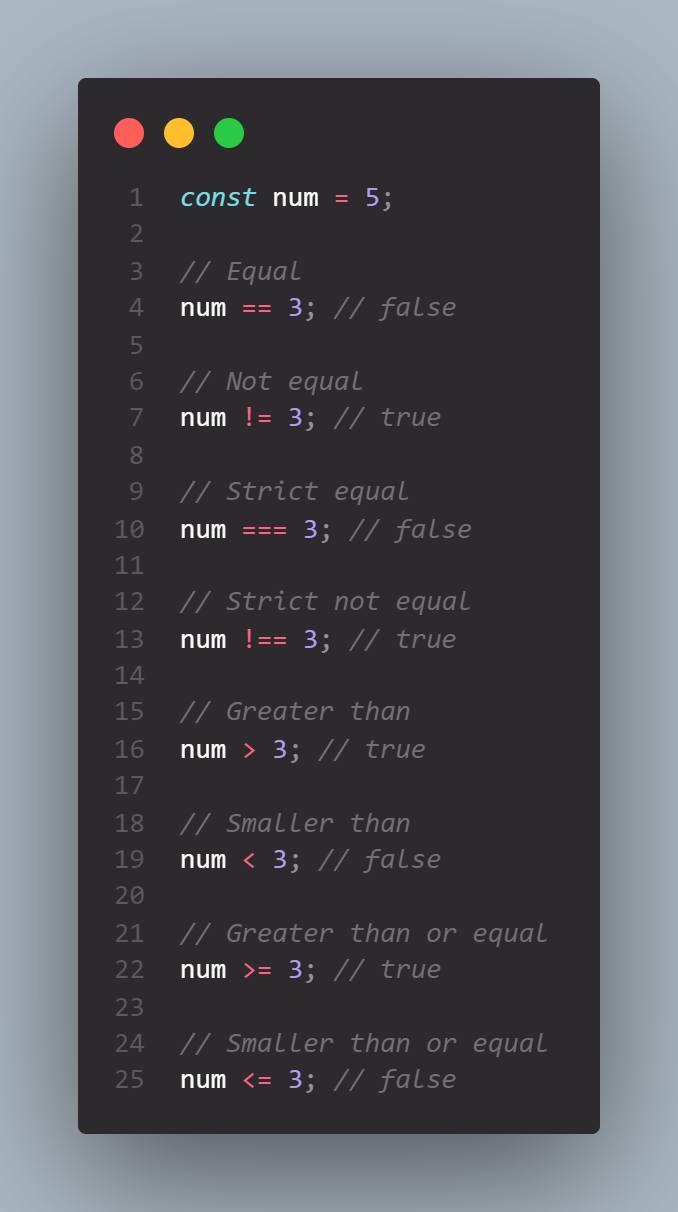# Basic Operators

JavaScript operators allows us to transform values or combine multiple values and really do all kinds of work with values. And there are many categories of operators like mathematical operators, comparison operators, logical operators, assignment operators, and many more.

Let's look at some of these types of operators.

## Mathematical / Arithmetic operators

Arithmetic operators are used to perform arithmetic on numbers.

Unary operation: an operation with only one operator.

Binary operator: an operation that operates on two operands and manipulates them to return a result.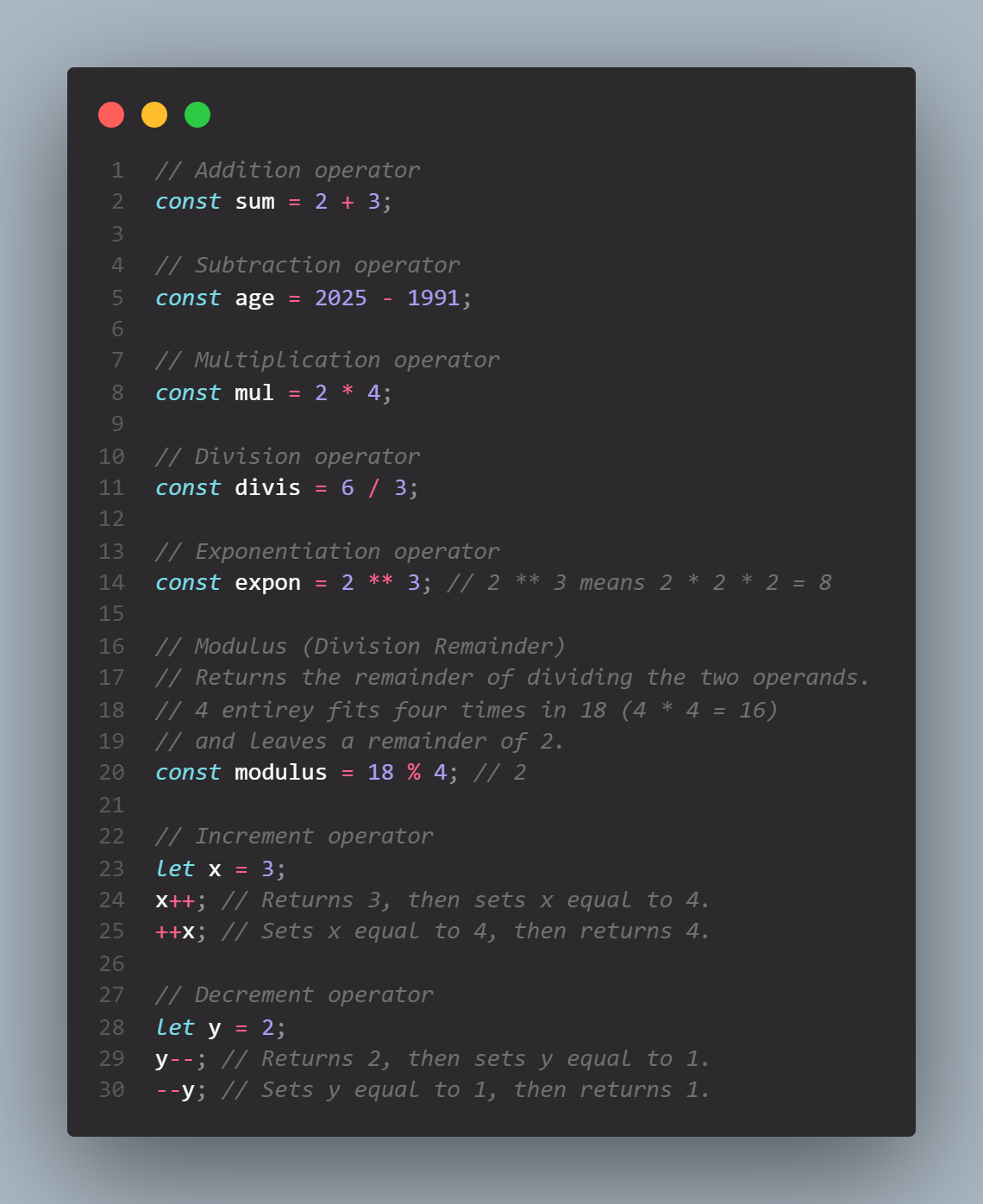## String operators

We can use the plus operator to join strings. Or in other words, to concatenate different strings.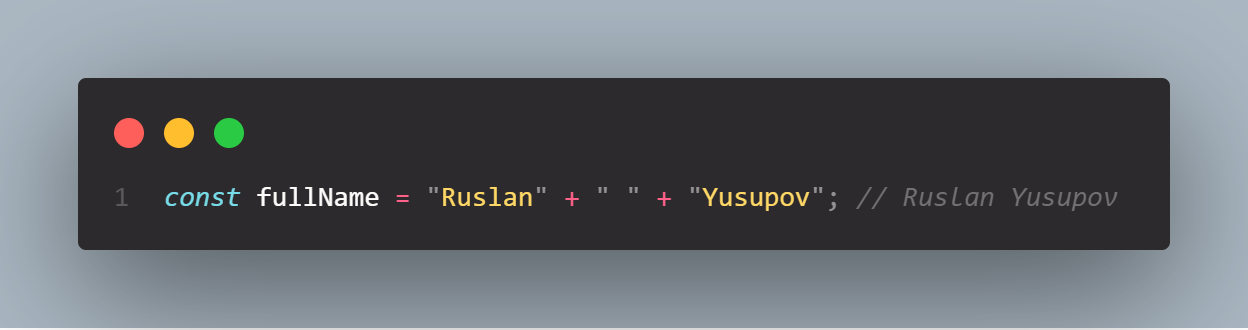## Type operators

typeof operator give us the type of the value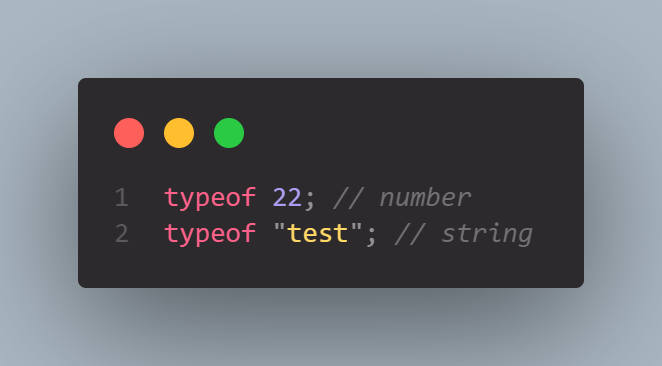## Assignment operators

Assignment operators assign values to JavaScript variables.

The most straightforward assignment operator is just the equal sign: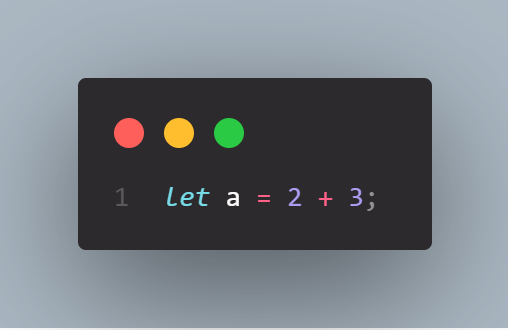And so this equal sign here is actually itself an operator. In this case x will be assigned 15 because the plus operator is executed before the assignment operator. And that's based on a couple of rules about operator precedence that we will learn in the next lesson.

There are more assignment operators: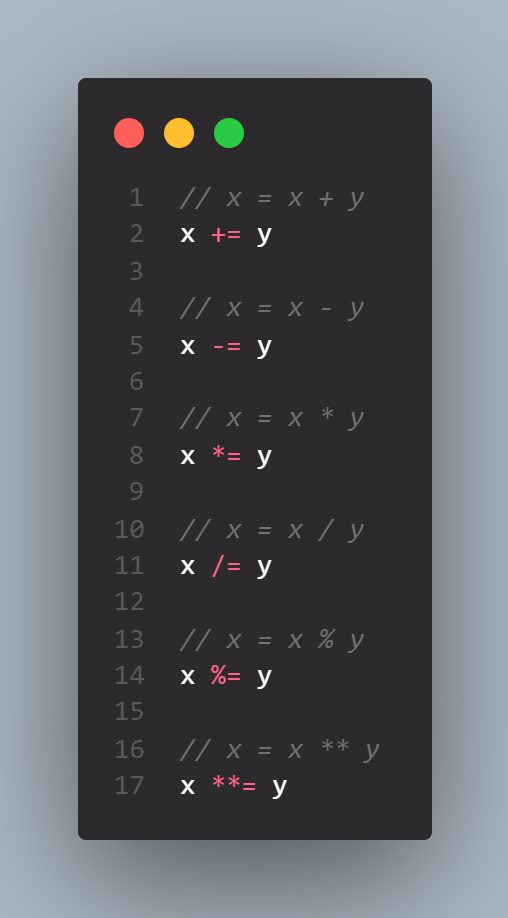## Comparison operators

We use comparison operators to produce boolean values. The result of comparison operator should be a boolean.

So, comparison operators compares its operands and returns a boolean based on whether the comparison is true or false.